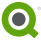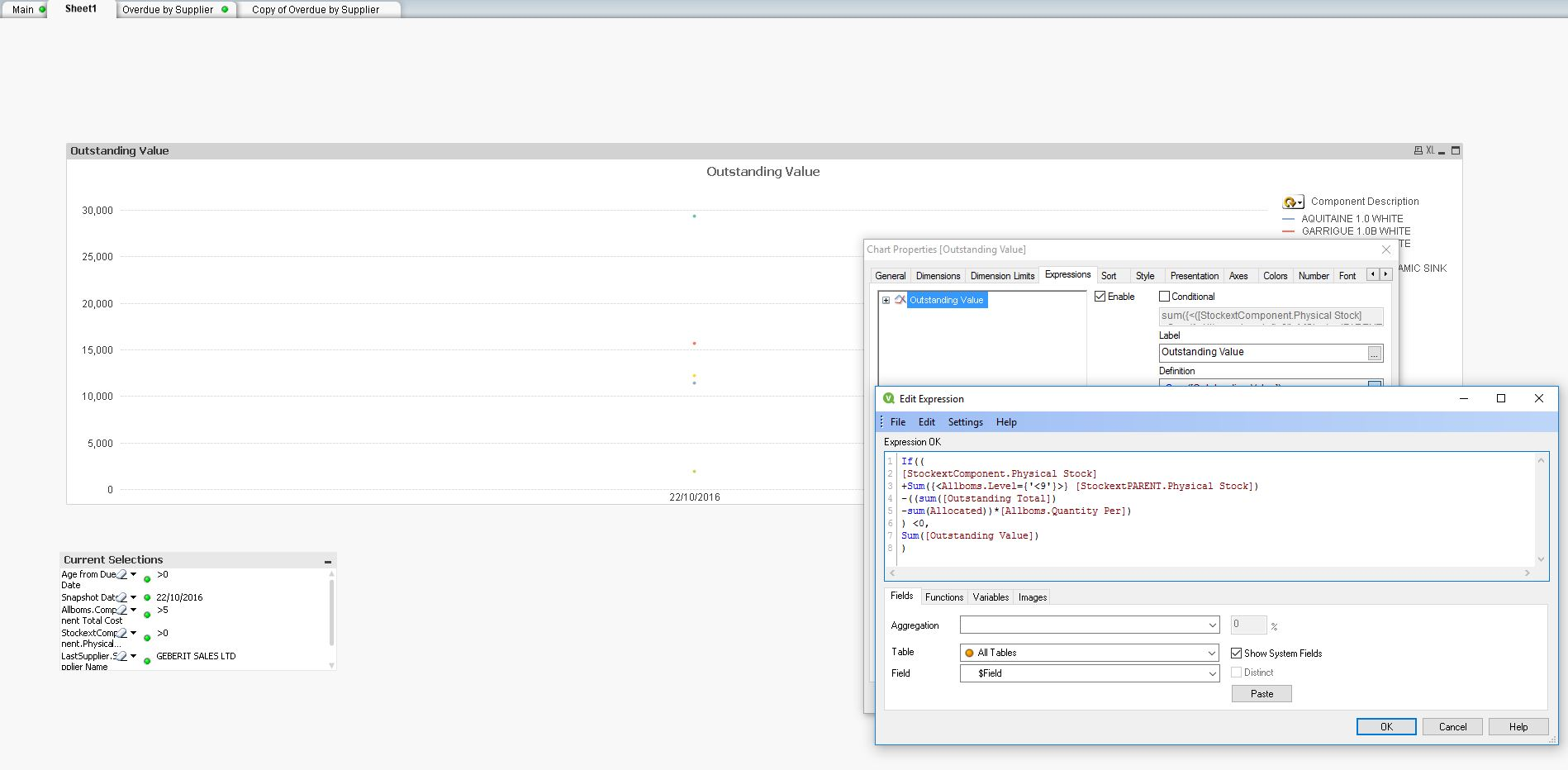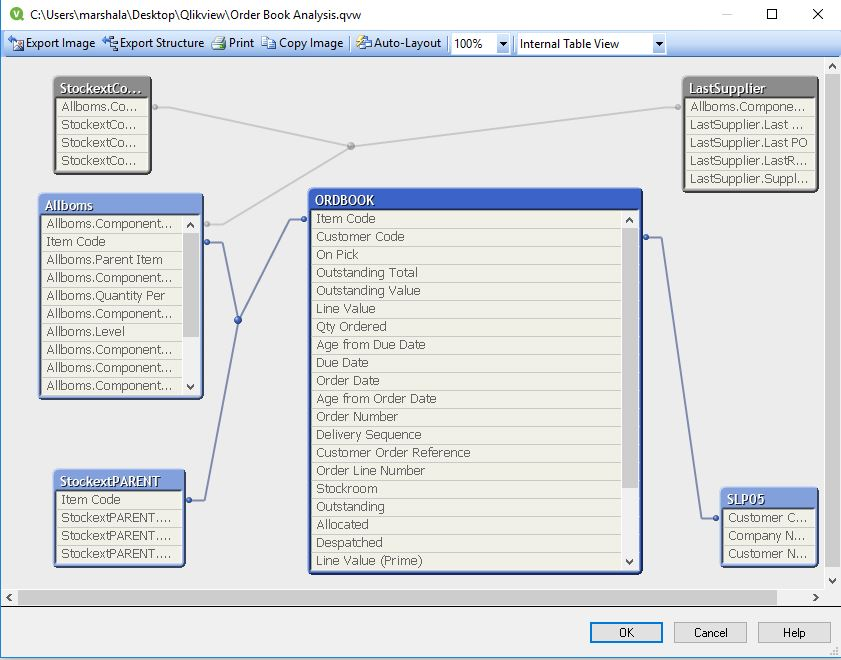# QlikView App Dev

Discussion Board for collaboration related to QlikView App Development.

Announcements
Our next Qlik Insider session will cover new key capabilities. Join us August 11th REGISTER TODAY
cancel
Showing results for
Did you mean:Not applicable

## Issue with set modifier expression... I can't figure it out :)

Hi Guys

So I'm graphing overdue stock by supplier name. I need the outstanding value to be totalled up only when we are short on stock. The expression for that is:

sum({<([StockextComponent.Physical Stock]

+Sum({<Allboms.Level={'<9'}>} [StockextPARENT.Physical Stock])

-((sum([Outstanding Total])

-sum(Allocated))*[Allboms.Quantity Per])))={'<0}>'} [Outstanding Value]

I'm getting "Error in set modifier expression" and the underlined part here is underlined in red.

Basically I want to do this formula:

[StockextComponent.Physical Stock]

+Sum({<Allboms.Level={'<9'}>} [StockextPARENT.Physical Stock])

-((sum([Outstanding Total])

-sum(Allocated))*[Allboms.Quantity Per])

And when it's <0 sum up the quantity of outstanding value.

Is that possible to do? or have I just got my brackets all in the wrong places throwing it out?

Thanks a lot for your help.

1 Solution

Accepted SolutionsMVP

Not sure if I understand 100%, but here is a suggestion:

You can use an expression search to filter the items in your set analysis, like

Sum(

{<

YourItemField = {"=([StockextComponent.Physical Stock]

+Sum({<Allboms.Level={'<9'}>} [StockextPARENT.Physical Stock])

-((sum([Outstanding Total])

-sum(Allocated))*[Allboms.Quantity Per])

) <0"}

>}

[Outstanding Value])

9 RepliesCreator II

It looks like you have an apostrophe in the wrong place on the last line. Does this work?

sum({<([StockextComponent.Physical Stock]

+Sum({<Allboms.Level={'<9'}>} [StockextPARENT.Physical Stock])

-((sum([Outstanding Total])

-sum(Allocated))*[Allboms.Quantity Per])))={'<0}'>} [Outstanding Value]Not applicable
Author

Ooops yeah, well spottedIt still doesn't work though, I think I changed it around that many times that was just a typo on the last attemptsum({<([StockextComponent.Physical Stock]

+Sum({<Allboms.Level={'<9'}>} [StockextPARENT.Physical Stock])

-((sum([Outstanding Total])

-sum(Allocated))*[Allboms.Quantity Per])))={'<0'}>} [Outstanding Value]

This underlined part still has error in expression...Not applicable
Author

Still struggling with this working. Anyone else with any idea's?

ThanksMaster

If a quick glance (highlighted in red)

sum({<([StockextComponent.Physical Stock]

+Sum({<Allboms.Level={'<9'}>} [StockextPARENT.Physical Stock])

-((sum([Outstanding Total])

-sum(Allocated))*[Allboms.Quantity Per])))={'<0'}>} [Outstanding Value]

1. No closing parenthesis

2. It is not clear what is being searched for is less than zero. If this is a calculation, and not a field, multiplication must also go into the set with zero.

3. In calculations, I would use double quotes instead of single {"= ..."}

Regards,

AndreyMVP

The set modifiers can only be applied to fields, not expressions. Just like you can only make selections on field values.

Maybe try

If(

Rangesum(

[StockextComponent.Physical Stock],Sum({<Allboms.Level={'<9'}>} [StockextPARENT.Physical Stock]),

-(Rangesum(sum([Outstanding Total]),-sum(Allocated))*[Allboms.Quantity Per])

) <0,

Sum([Outstanding Value])

)

edit: corrected parentheses and added another RangesumNot applicable
Author

Thanks for your answers guys. Very much appreciated!!I certainly hadn't realized "The set modifiers can only be applied to fields, not expressions"

The rangesum here didn't get me any values, but I also hadn't realized you can use IF statements. So just using the IF statement has gotten me further!

Andrey, I'm wanting to sum up the outstanding value where the stock level for the item is <0 when taking into account demand from orders on our system which is calculated as:

"sum({<([StockextComponent.Physical Stock]

+Sum({<Allboms.Level={'<9'}>} [StockextPARENT.Physical Stock])

-((sum([Outstanding Total])

-sum(Allocated))*[Allboms.Quantity Per])))"

At the moment I can't graph it because the above calculation is in a table box, it gives me positive and negative values. I manually select the negative values. Which is fine for the tables. Trouble is, if I select more than 1 date to graph it, I can no longer select negatives in the table because they're summing up for the entire date period so the data becomes nonsensical. I need the formula to do that itself in the graph expression for each date. This is where I'm now struggling.

If((

[StockextComponent.Physical Stock]

+Sum({<Allboms.Level={'<9'}>} [StockextPARENT.Physical Stock])

-((sum([Outstanding Total])

-sum(Allocated))*[Allboms.Quantity Per])

) <0,

Sum([Outstanding Value])

)

This now works for the graphing but only when I have Component Item as my dimension. I need to have Supplier as my dimension as a supplier overdue analysis.

How do I get this to work for Supplier?

Attached some images so you can better see what's going on.

Thanks!Not applicable
Author

I'm guessing the reason I get no graph data when I set the dimension to "Supplier" is because the net stock level for the supplier is >0. It'll be doing that calculation over all items combined so rather than what I need (The value of the items <0) It's adding up all items and giving me suppliers where the nett is <0 ... which is none of them!

I need to force the expression over item level?

ThanksMVP

Not sure if I understand 100%, but here is a suggestion:

You can use an expression search to filter the items in your set analysis, like

Sum(

{<

YourItemField = {"=([StockextComponent.Physical Stock]

+Sum({<Allboms.Level={'<9'}>} [StockextPARENT.Physical Stock])

-((sum([Outstanding Total])

-sum(Allocated))*[Allboms.Quantity Per])

) <0"}

>}

[Outstanding Value])Not applicable
Author

Thanks Stefan

That does seem to do exactly what I am wanting!

I'll need to do some data spot checking to triple check but that does appear to have nailed it

Thanks